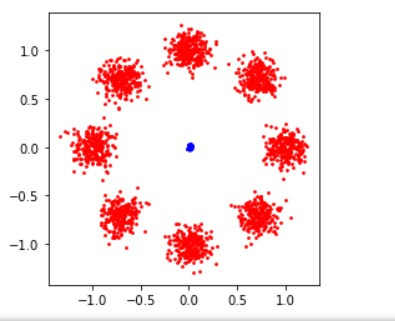# Wrong training result with changing forward function in VAE

I have a VAE model with the below forward function and loss function:

``````def forward(self, x):
mu, logvar = self.encode(x.view(-1, 2))
z = self.reparameterize(mu, logvar)
return self.decode(z), mu, logvar

def loss_function(recon_x, x, mu, logvar):
L2 = torch.mean((recon_x-x)**2)
KLD = -0.5 * torch.mean(1 + logvar - mu.pow(2) - logvar.exp())
return L2 + KLD
``````

the result of training for the multivariate gaussian dataset is similar to the photo below:To do something, I changed the forward and loss function as follows

``````def forward(self, x):
mu, logvar = self.encode(x.view(-1, 2))
z = self.reparameterize(mu, logvar)
q0 = torch.distributions.normal.Normal(mu, (0.5 * logvar).exp())
prior = torch.distributions.normal.Normal(0., 1.)
log_prior_z = prior.log_prob(z).sum(-1)
log_q_z = q0.log_prob(z).sum(-1)
return self.decode(z), log_q_z - log_prior_z

def loss_function(recon_x, x, KL):
L2 = torch.mean((recon_x-x)**2)
return L2 + KL
``````

but the training result is as followsHow do I make these changes to get the same result?